DAY 4
0
AI & Data

# 前言

「眼前的黑不是黑，你說的白是什麼白」-- 你是我的眼(蕭煌奇)

• 什麼是灰度直方圖(Histogram)：
X軸為像素值(0 ~ 255)，Y軸為該值的數量。

• 灰度直方圖的特性：
若圖片全黑(像素為0)，則直方會集中在圖的左側，代表圖片太暗了。

• 觀察照片(angry)：
因為下圖(MDFK)較上圖暗，所以直方圖的bin偏向左邊。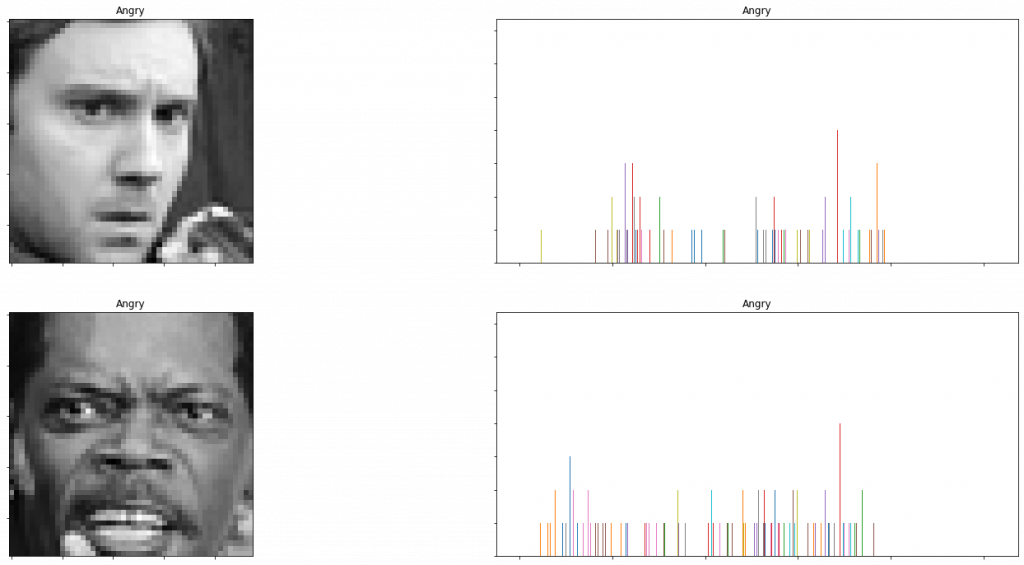• 觀察照片(neutral)：
因為上圖(女人)各個灰度值發生較平均，所以直方圖的bin呈現平均分散的狀態，
而下圖(嬰兒)黑白分明，少有中間灰色，所以直方圖的bin集中在兩側，中間只有零星幾個bin。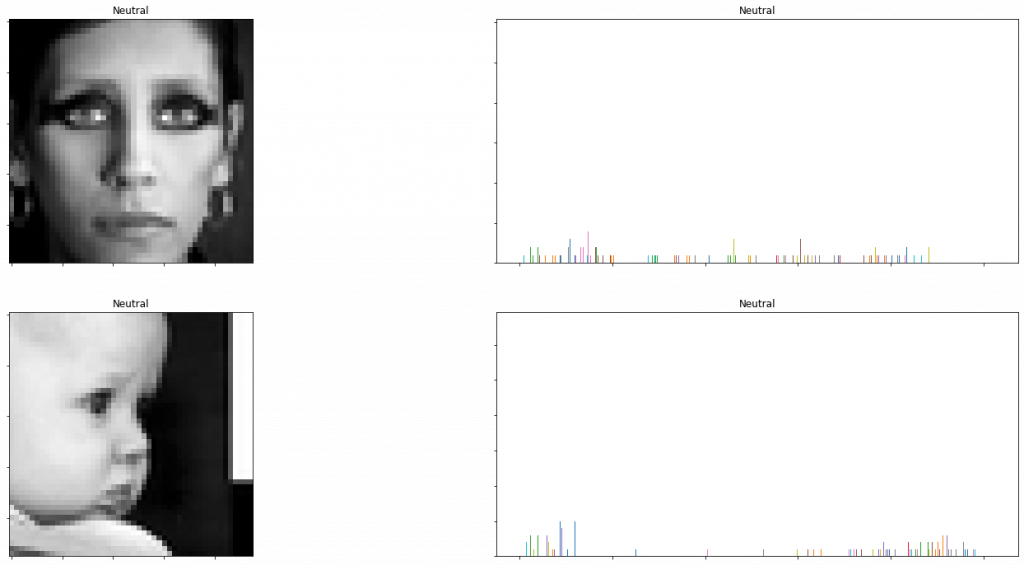## 1. 原始圖片

``````idx = 12
gray_img = X_train[idx][:, :, 0].astype("uint8")
gray_img = cv2.resize(gray_img, (600, 600))
plt.figure(figsize=(5, 5))
plt.imshow(gray_img, cmap='gray')
``````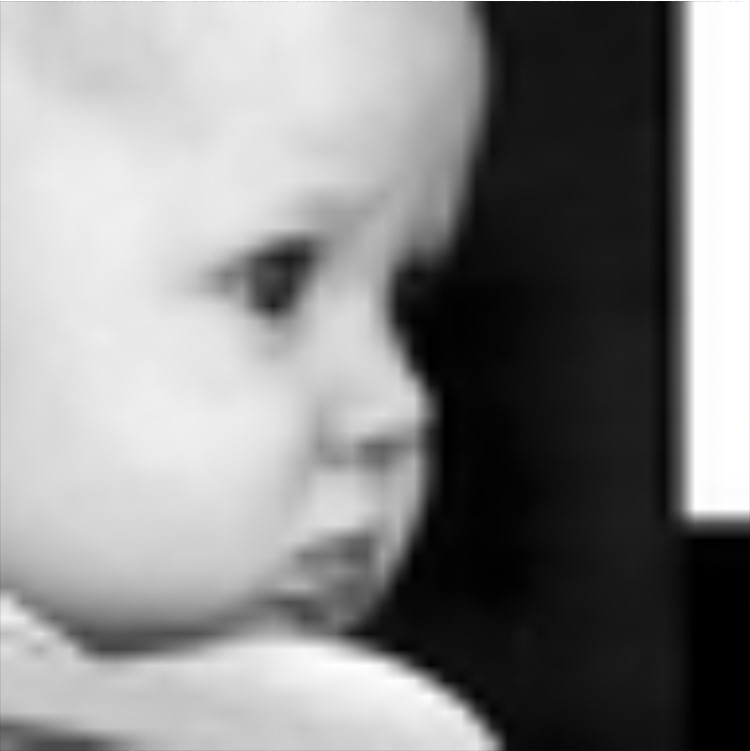## 2. 直方圖均衡化(Histogram Equalization)

``````equalized_img = cv2.equalizeHist(gray_img)
cv2.imshow("equal_image", equalized_img)
``````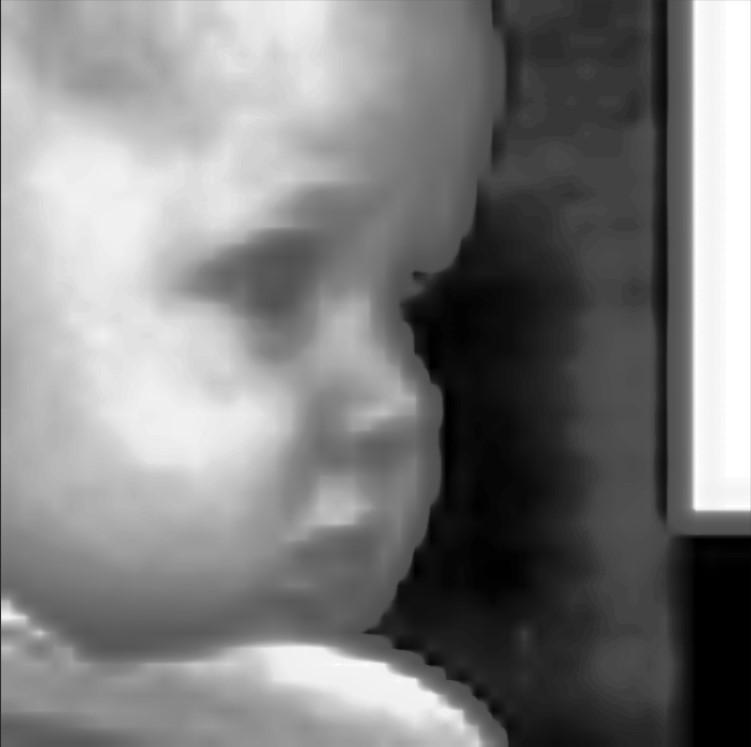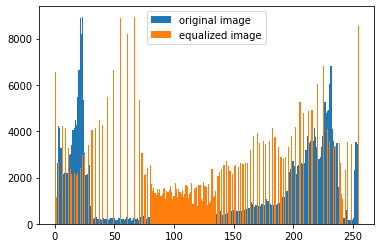``````clahe = cv2.createCLAHE(clipLimit=2)
clahe_img = clahe.apply(gray_img)
cv2.imshow("clahe_img", clahe_img)
``````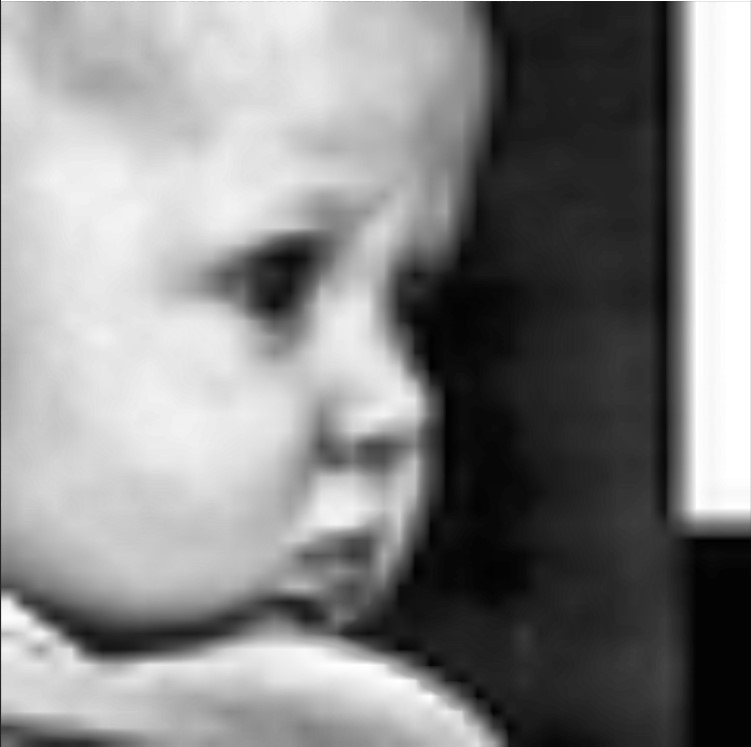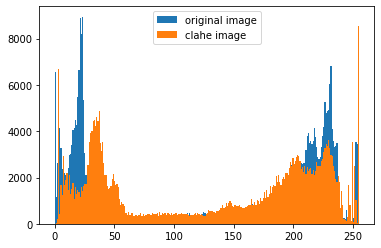## 結語

1. 用數值方法將圖片的size變大(實際上就是cv2.resize，要用GAN也可以)
2. 增加圖片對比度(CLAHE)